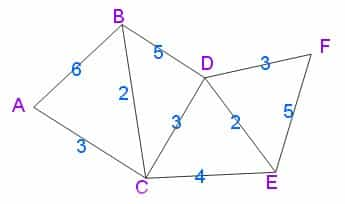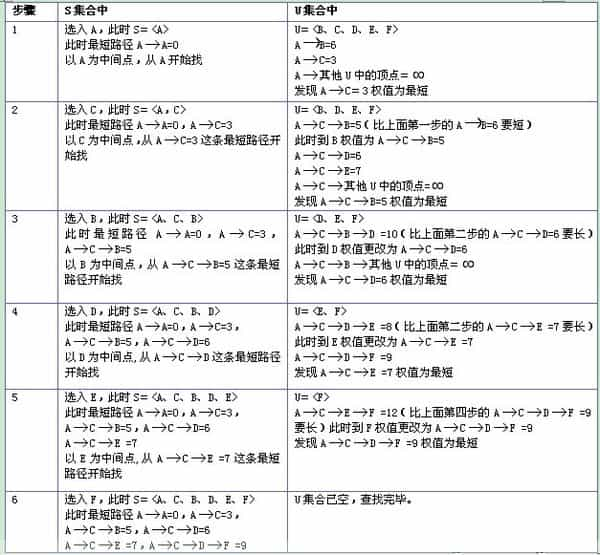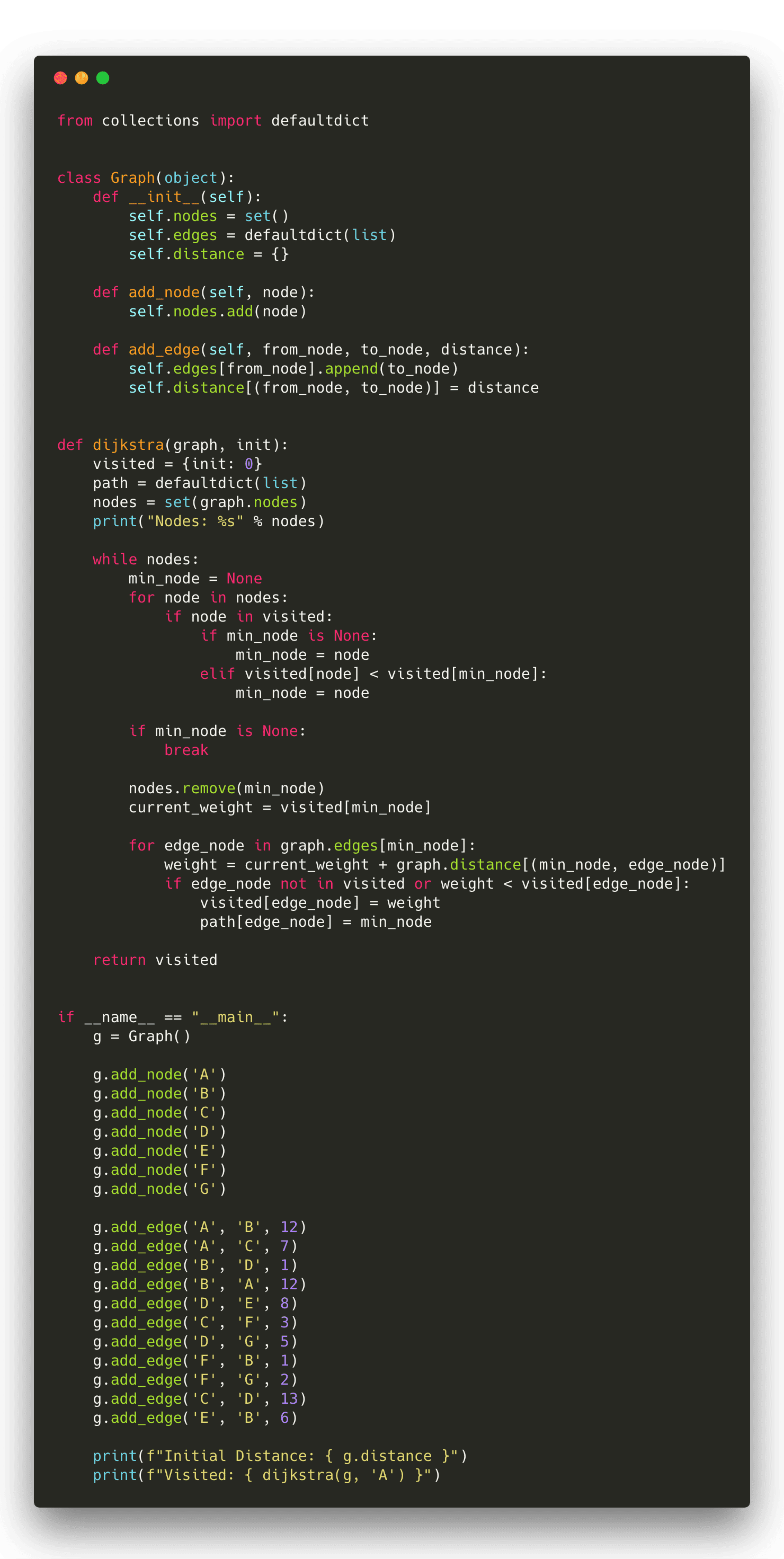# Dijkstra Algorithm

### Intro

Dijkstra’s algorithm is an algorithm for finding the shortest paths between nodes in a graph, which may represent, for example, road networks. It was conceived by computer scientist Edsger W. Dijkstra in 1956 and published three years later.

### Steps

Illustration of Dijkstra’s algorithm finding a path from a start node (lower left, red) to a goal node (upper right, green) in a robot motion planning problem. Open nodes represent the “tentative” set (aka set of “unvisited” nodes). Filled nodes are visited ones, with color representing the distance: the greener, the closer. Nodes in all the different directions are explored uniformly, appearing more-or-less as a circular wavefront as Dijkstra’s algorithm uses a heuristic identically equal to 0. Let the node at which we are starting be called the initial node. Let the distance of node Y be the distance from the initial node to Y. Dijkstra’s algorithm will assign some initial distance values and will try to improve them step by step.

1. Mark all nodes unvisited. Create a set of all the unvisited nodes called the unvisited set.
2. Assign to every node a tentative distance value: set it to zero for our initial node and to infinity for all other nodes. Set the initial node as current.
3. For the current node, consider all of its unvisited neighbors and calculate their tentative distances through the current node. Compare the newly calculated tentative distance to the current assigned value and assign the smaller one. For example, if the current node A is marked with a distance of 6, and the edge connecting it with a neighbor B has length 2, then the distance to B through A will be 6 + 2 = 8. If B was previously marked with a distance greater than 8 then change it to 8. Otherwise, keep the current value.
4. When we are done considering all of the neighbors of the current node, mark the current node as visited and remove it from the unvisited set. A visited node will never be checked again.
5. Move to the next unvisited node with the smallest tentative distances and repeat the above steps which check neighbors and mark visited.
6. If the destination node has been marked visited (when planning a route between two specific nodes) or if the smallest tentative distance among the nodes in the unvisited set is infinity (when planning a complete traversal; occurs when there is no connection between the initial node and remaining unvisited nodes), then stop. The algorithm has finished.
7. Otherwise, select the unvisited node that is marked with the smallest tentative distance, set it as the new “current node”, and go back to step 3.### Code

https://github.com/Flowerowl/basic_algorithm/blob/master/graph/dijkstra.py